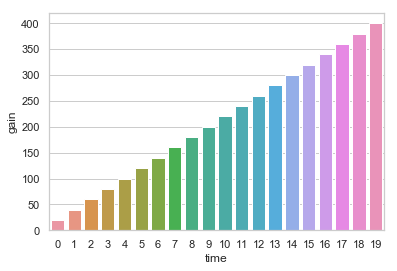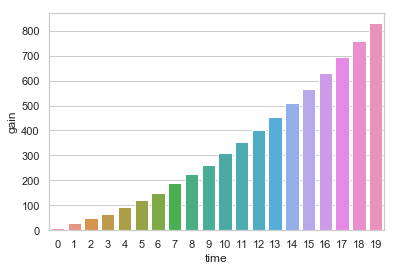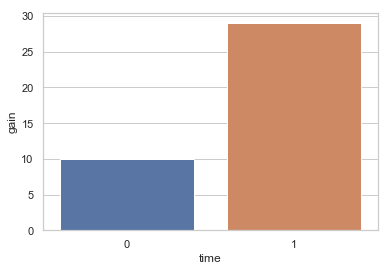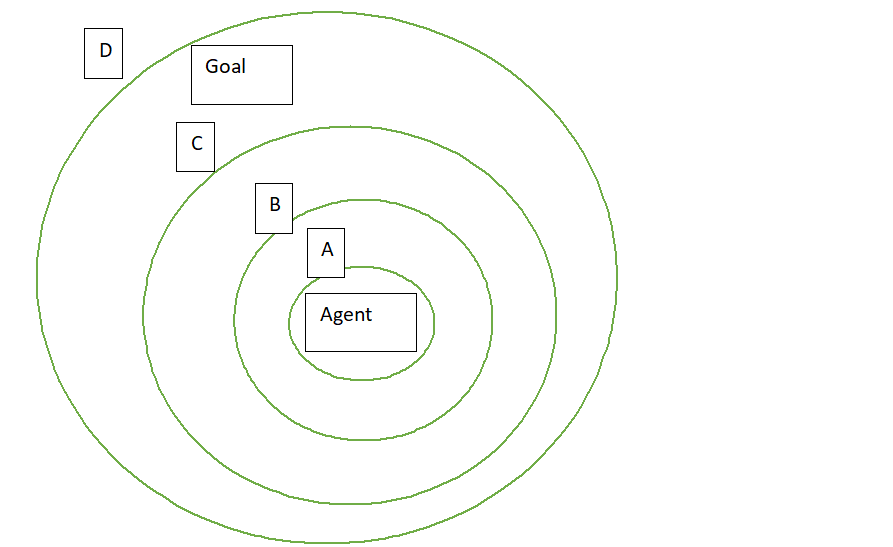# What is exploration–exploitation dilemma?

```import pandas as pdimport seaborn as sns
```
```class agent_1:gain = 0exploit = 10explore = 30exploreFactor = 0.3score = 0class agent_2:gain = 0exploit = 20explore = 30exploreFactor = 0.3score = 0
```
```agent_1 = agent_1()agent_2 = agent_2()agent_1_gainList = []agent_2_gainList = []time = []
```
```for i in range(20):agent_1.gain += agent_1.exploit agent_2.gain += agent_2.exploitagent_1_gainList.append(agent_1.gain)agent_2_gainList.append(agent_2.gain)time.append(i)if i % 3 == 0:agent_1.exploit += agent_1.explore * agent_1.exploreFactorif i % 5 == 0:if agent_1.gain > agent_2.gain:agent_1.score += 1elif agent_2.gain > agent_1.gain:agent_2.score += 1else:passprint(agent_1.score,agent_2.score)
```
```Agent_1GainTable = {'time':time,'gain':agent_1_gainList}Agent_2GainTable = {'time':time,'gain':agent_2_gainList}df_1 = pd.DataFrame.from_dict(Agent_1GainTable)df_2 = pd.DataFrame.from_dict(Agent_2GainTable)
```
```sns.set(style="whitegrid")ax = sns.barplot(x="time", y="gain", data=df_1)
```

So in every 3 time, we explore the environment in agent 1. When we explore, in learning or from beneficial source, learning could be always helpful and beneficial. That’s why we add some value to exploit variable.

In the end we can see that gain of agent_2and gain of agent_1with 50 iterate we reach agent_1: 9 and agent_2: 1

with 2 iterate we reach agent_1: 0 and agent_2: 1Although we have nice increment at exploring, in little time, other agent could be successfull. That’s why, we can make analogy that, even the other agents or agent is more sophisticated, the unadvanced one could be more successfull in little time.

### The Explore Function

We can add two different explore function to agents. The one way to measure explore benefit is measuring change of benefit of explore gain data. We can predict this data with machine learning algorithm or time series.

We can see the functional body as this:

```import pandas as pdimport seaborn as snsimport math
```
```class agent_1:gain = 0exploit = 10explore = 30exploreFactor = 0.3score = 0def explorefunction(self):self.exploit += self.explore ** 3 - 28*self.explore**2 - 250class agent_2:gain = 0exploit = 10explore = 30exploreFactor = 0.3score = 0def explorefunction(self):self.exploit += math.exp(self.explore) - self.explore**15
```
```agent_1 = agent_1()agent_2 = agent_2()agent_1_gainList = []agent_2_gainList = []time = []
```
```for i in range(100):agent_1.gain += agent_1.exploit agent_2.gain += agent_2.exploitagent_1_gainList.append(agent_1.gain)agent_2_gainList.append(agent_2.gain)time.append(i)if i % 3 == 0:agent_1.explorefunction()agent_2.explorefunction()if i % 5 == 0:if agent_1.gain > agent_2.gain:agent_1.score += 1elif agent_2.gain > agent_1.gain:agent_2.score += 1else:passprint(agent_1.score,agent_2.score)
```

Score is agent_1 : 19 agent_2 : 0

According to the functions agent 1 or agent 2 is winning.

### Determining Explore Function

The hard part of modeling the total gain structure, we don’t know the general environment and we need a functional body to determine explore addition to total gain. In this problem, we should able to model the information sources. For example, one agent must understand the true need of supervisor or transform these information gain into the reward of supervisor.

For instance, when we learn to walk, the supervisor is nature the physical law itself. When we can walk properly, nature rewards us. Indeed, we should able to distinguish the correct way of walking and not walking.

Thus, generally the supervisor in games or nature is the mathematical structure of games or nature. We should able to model the supervisor and transform it a usable structure in model. This is not easy task as said and can vary in every movement and environment.

If we can model the information source structure, we should do. Otherwise we must explore with no information.

### Exploration SpaceFor example , we have a space like this. Yeah, first thing to do is to configure the space of agent and the goal. This can be done by supervised which is another issue. When we configure the environment space of exploration, we should reach our purpose or goal with minimum state with reinforcement learning.

for Example a pathaway to goal could be like this

Agent → A(A¹ → A² →A⁴ →A³) → B(B² → B³ → B¹ →B⁴) → C(C …) → Goal

We have several problem here.

Naturally, there can be paths to goal which jumps from A to B or A to C and ther e can be states from B to D and so on.

Some clusters may not be inside of another cluster fully.Thus, we could classify these cluster types according to their behaviours.

### Information gain in graphs

We can say that when we travel on nodes in clusters, the degree of node could be reward for search function. if we look 3 nodes and which has most degree we select this node to search on. Because naturally, this node has more information than others. We want to maximize our information in fastest way. Thus, we select the best information nodes and theorically, when we explore this way, we could find the goal node fastest in natural structures.